# Adding And Subtracting Decimals Worksheets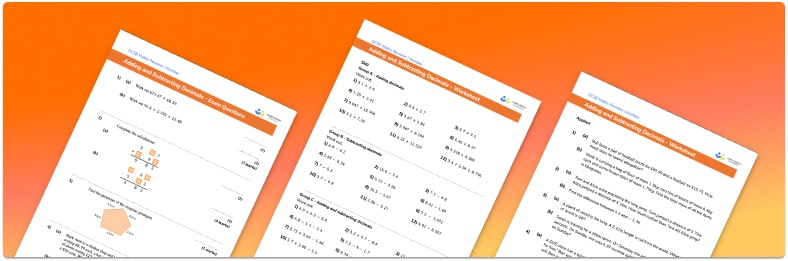• This field is for validation purposes and should be left unchanged.

You can unsubscribe at any time (each email we send will contain an easy way to unsubscribe). To find out more about how we use your data, see our privacy policy.

• Section 1 of the adding and subtracting decimals worksheet contains 36 skills-based adding and subtracting decimals questions, in 3 groups to support differentiation
• Section 2 contains 4 applied adding and subtracting decimals questions with a mix of word problems and deeper problem solving questions
• Section 3 contains 4 foundation and higher level GCSE exam style adding and subtracting decimals questions
• Answers and a mark scheme for all adding and subtracting decimals questions are provided
• Questions follow variation theory with plenty of opportunities for students to work independently at their own level
• All questions created by fully qualified expert secondary maths teachers
• Suitable for GCSE maths revision for AQA, OCR and Edexcel exam boards

### Adding and subtracting decimals at a glance

If a number has a part that is not whole, it is called a decimal number. We write the whole number part, followed by a decimal point, then tenths, hundredths, thousandths, and so on. It is important that students can recognise the decimal place value of each column on a place value chart – for example, know that 0.6 represents 6 tenths. Ordering decimals also requires students to apply this knowledge of place value.

Decimal addition follows the same method as integer addition. We write the numbers in columns according to their place value: units under units, tens under tens and so on. The decimal points should be lined up, as should the values (tenths, hundredths etc) after the decimal points, and any gaps filled with zeroes. Working from the column furthest right (so with the smallest place value), add each column of digits, carrying into the next column if necessary.

To carry out a decimal subtraction, we line up the numbers as described for addition, ensuring that the first number in the calculation is on the top. We then subtract each column of digits, borrowing from the next column if needed as we would in integer subtraction.

These skills are then extended further to dealing with decimal multiplication and decimal division, including long division methods.

Looking forward, students can then progress to additional number worksheets, for example an order of operations worksheet or a fraction subtraction worksheet.For more teaching and learning support on Number our GCSE maths lessons provide step by step support for all GCSE maths concepts.

## Related worksheets

Decimals Worksheets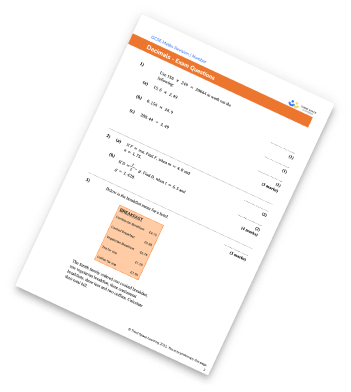Multiplying And Dividing Decimals Worksheet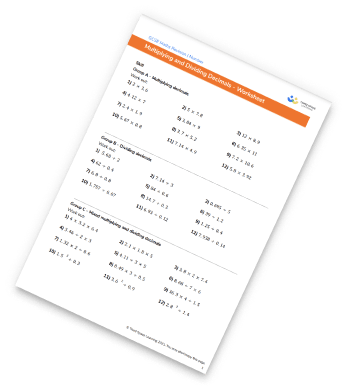Multiplying Fractions Worksheet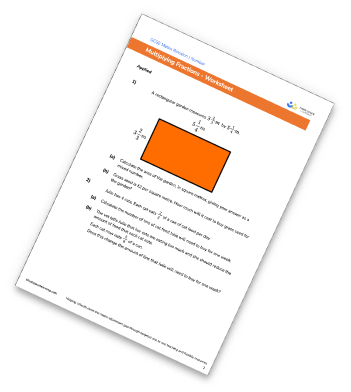Rational And Irrational Numbers Worksheet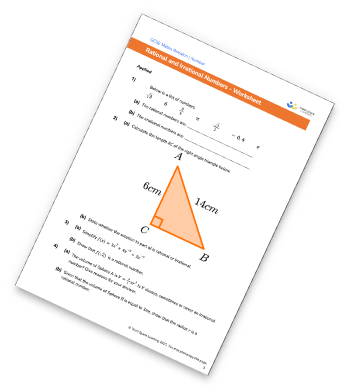## Do you have KS4 students who need more focused attention to succeed at GCSE?There will be students in your class who require individual attention to help them succeed in their maths GCSEs. In a class of 30, it’s not always easy to provide.

Help your students feel confident with exam-style questions and the strategies they’ll need to answer them correctly with our dedicated GCSE maths revision programme.

Lessons are selected to provide support where each student needs it most, and specially-trained GCSE maths tutors adapt the pitch and pace of each lesson. This ensures a personalised revision programme that raises grades and boosts confidence.

Find out more